• 参数方程求导： syms t x = cos(t)^3 y = sin(t)^3 dx1dt = diff(x,t); % 一阶导数 dy1dx = diff(y,t)/dx1dt % 二阶导数 dy2d2x = diff(dy1dx,t)/dx1dt % 3阶导数 dy3d3x = diff(dy2d2x,t)/dx1dt ②求不定积分 单...
①参数方程求导：
syms  t
x =  cos(t)^3
y =  sin(t)^3
dx1dt = diff(x,t);
%  一阶导数
dy1dx = diff(y,t)/dx1dt
%  二阶导数
dy2d2x = diff(dy1dx,t)/dx1dt
%  3阶导数
dy3d3x = diff(dy2d2x,t)/dx1dt
②求不定积分
单变量被积函数表达式,f(x)
例1：syms x
int(f)
多变量被积函数表达式，f(x,y)  v为积分变量，是x或y
例2：syms x y;
int(f(x), v)
tips：ln(x)在matlab中输入：log(x)
二次根号下f在matlab中输入：f^0.5
e的x次方在在matlab中输入：exp(x)


展开全文matlab
• %参数方程求导的递归实现 % 调用格式： % y1 = paradiff(y, x, t, n) % 其中：y=f(t), x=g(t), t为参数, n为导数阶次 % % Examples: % 已知参数方程 y=sin(t)/(t+1)^3; x=cos(t)/(t+1)^3 % 求d3y/dx3 % MATLAB求解...
目录总述函数说明应用举例函数实现
总述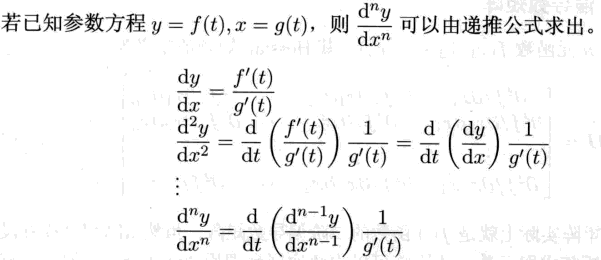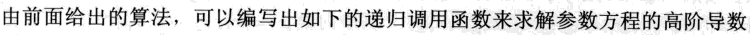函数说明
function result=paradiff(y, x, t, n)
%参数方程求导的递归实现
%  调用格式：
%    y1 = paradiff(y, x, t, n)
%  其中：y=f(t), x=g(t), t为参数, n为导数阶次
%
% Examples:
%  已知参数方程 y=sin(t)/(t+1)^3; x=cos(t)/(t+1)^3
%  求d3y/dx3
% MATLAB求解语句：
%  syms t; y=sin(t)/(t+1)^3; x=cos(t)/(t+1)^3;
%  [n,d]=numden(f);   %分离分子分母
%  F=simplify(n)/simplify(d)  %分子分母各自化简

应用举例
问题： 已知参数方程
$y=\frac{sint}{(t+1)^3}, x=\frac{cost}{(t+1)^3}$
求$d^3y/dx^3$
代码如下：
syms t
y=sin(t)/(t+1)^3; x=cos(t)/(t+1)^3;
[n,d]=numden(f);              % //分离分子分母
F=simplify(n)/simplify(d)     % //分子分母各自化简

最终结果为：函数实现
function result=paradiff(y, x, t, n)
if mod(n,1)~=0
error('n should positive integer, please correct')
else
if n==1
result = diff(y,t)/diff(x,t);
else
end
end


此函数源文件可前往下面网址下载：


展开全文matlab 数学建模
• 如果方程f(x,y)=0能确定y是x的函数，那么称这种方式表示的函数是隐函数。而函数就是指：在某一变化过程中，两个变量x、y，对于某一范围内的x的每一个值，y都有确定的值和它对应，y就是x的函数。这种关系一般用y=f(x)...
隐函数
如果方程f(x,y)=0能确定y是x的函数，那么称这种方式表示的函数是隐函数。而函数就是指：在某一变化过程中，两个变量x、y，对于某一范围内的x的每一个值，y都有确定的值和它对应，y就是x的函数。这种关系一般用y=f(x)即显函数来表示。f(x,y)=0即隐函数是相对于显函数来说的。
其实隐函数的知识并不难理解，我们以前学的因变量y在函数一边的叫做显函数；隐函数就是将y“隐藏”在一个式子里即和 自变量x在一边的函数。它的难点在于如何利用隐函数求导。接下来，我就和大家聊一聊隐函数的求导。
在做题的时候我们经常会听到“对x求导”的说法，这个就是我们往往不好理解的地方，知道如何处理x却不知道如何处理y，下面我就主要围绕这个来展开。举个例子：这个式子你当然可以将隐函数显化（就是先将隐函数转化成显函数，然后利用显函数进行求导）得出结果-⅔但是这样做题有些无法显化的函数就没法算了，所以我今天着重给大家讲一下它的通用解法：首先第一个x的导数是2这个大家都知道，可是y咱们也需要处理，怎么处理？无论函数是否可以显化，x是自变量y是因变量（y是关于x的函数）是一定的吧。之后呢？就是说在y这里对x求导就是对含有x的小函数求导（我这里说小函数是为了和原来的隐函数区分一下的），这回结果不就是y′(即dy/dx)吗？这么一变形，就出现了2+3dy/dx=0导数就是-2/3尽管答案一样但是这种思考方式就会在做题的时候给你带来好处。
那么咱们换一个有点难度的，带平方的该如何计算呢？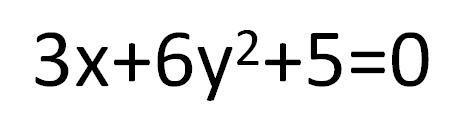求x=-3/5时的导数。
那么我们就可以计算了：3+62ydy/dx=0，那么dy/dx=-3/12y由于我是随便出的例子，经过计算此时y等于零，导数不存在。但是无论什么题都是这么是思考的。
最后还有一个对数求导法，是用来求幂指函数的。举个例子，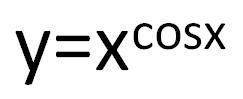我们给它两边取对数那么左边就会变成lny右边就是lnx^cosx，根据我们高中学的定理，右边还等于cosxlnx所以，就有lny=cosxlnx,之后咱们再根据求导的法则来求它。
左边就是还要把lny视作一个整体来看，右边要注意的事导数相乘时的运算。
求导法则
对于一个隐函数已经确定存在且可导的情况下，我们可以用复合函数求导的链式法则来进行求导。在方程左右两边都对x进行求导，由于y其实是x的一个函数，所以可以直接得到带有 y’ 的一个方程，然后化简得到 y’ 的表达式。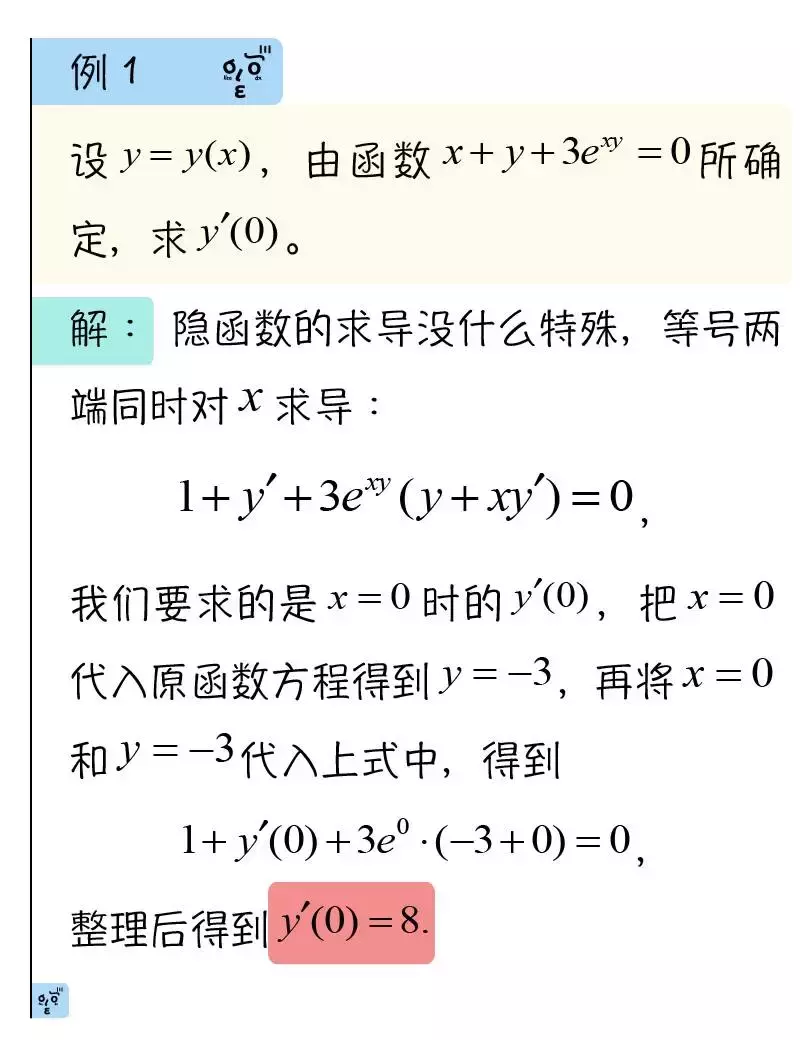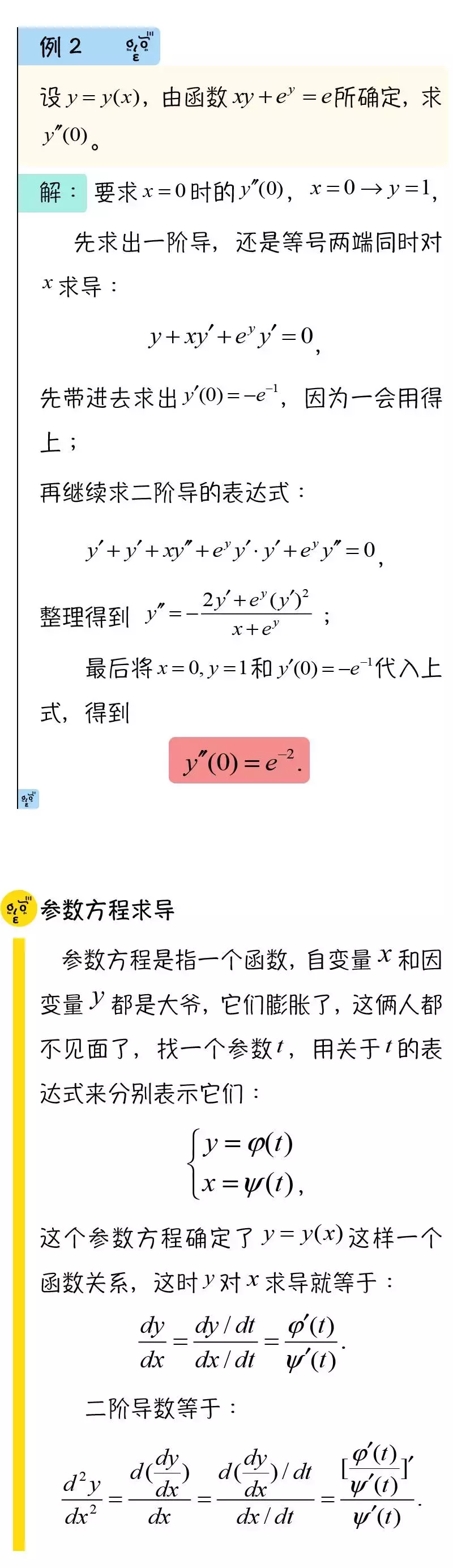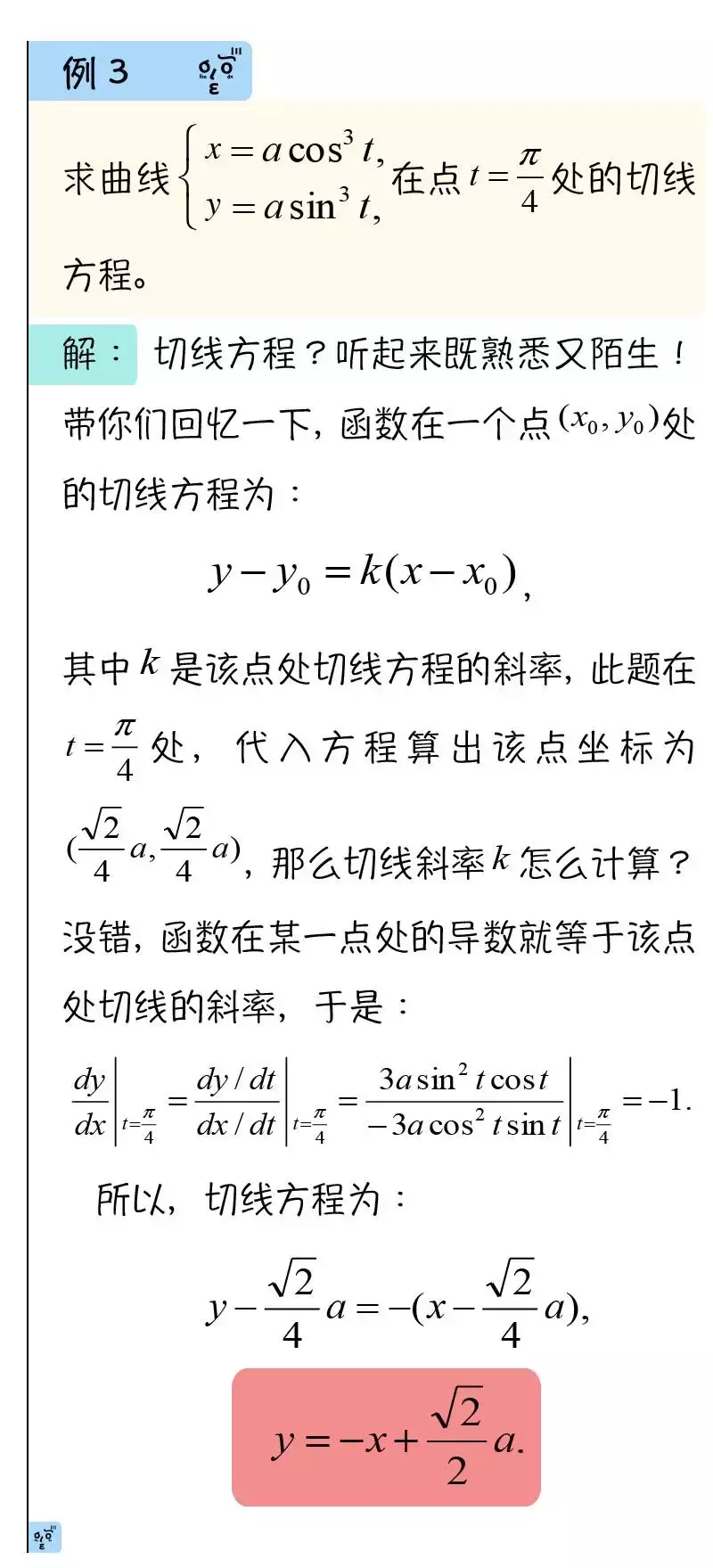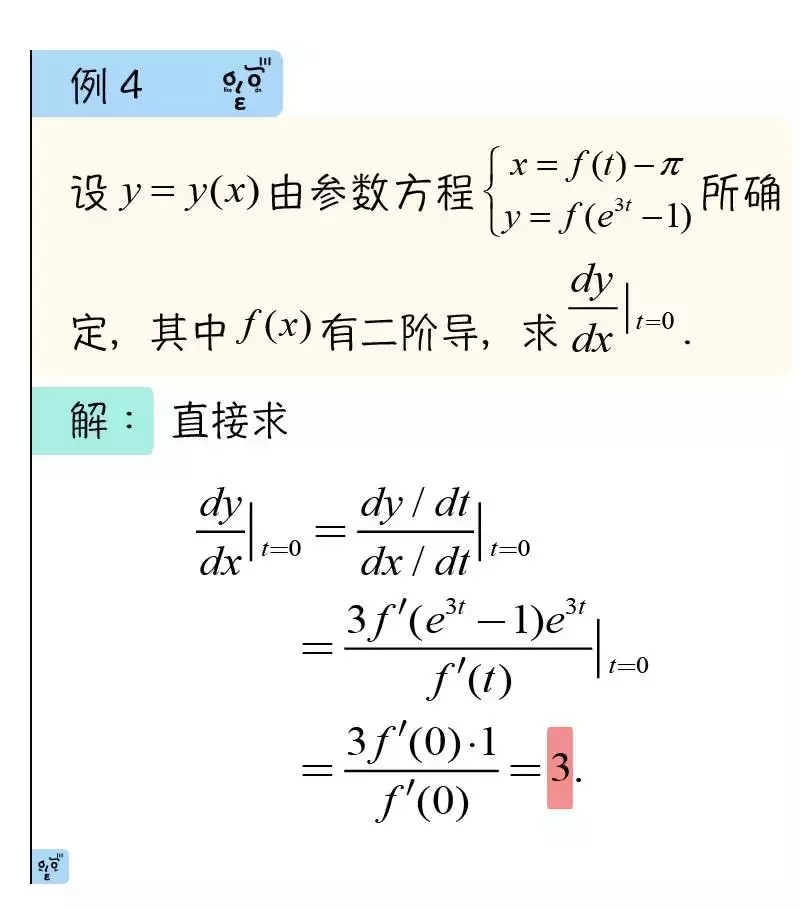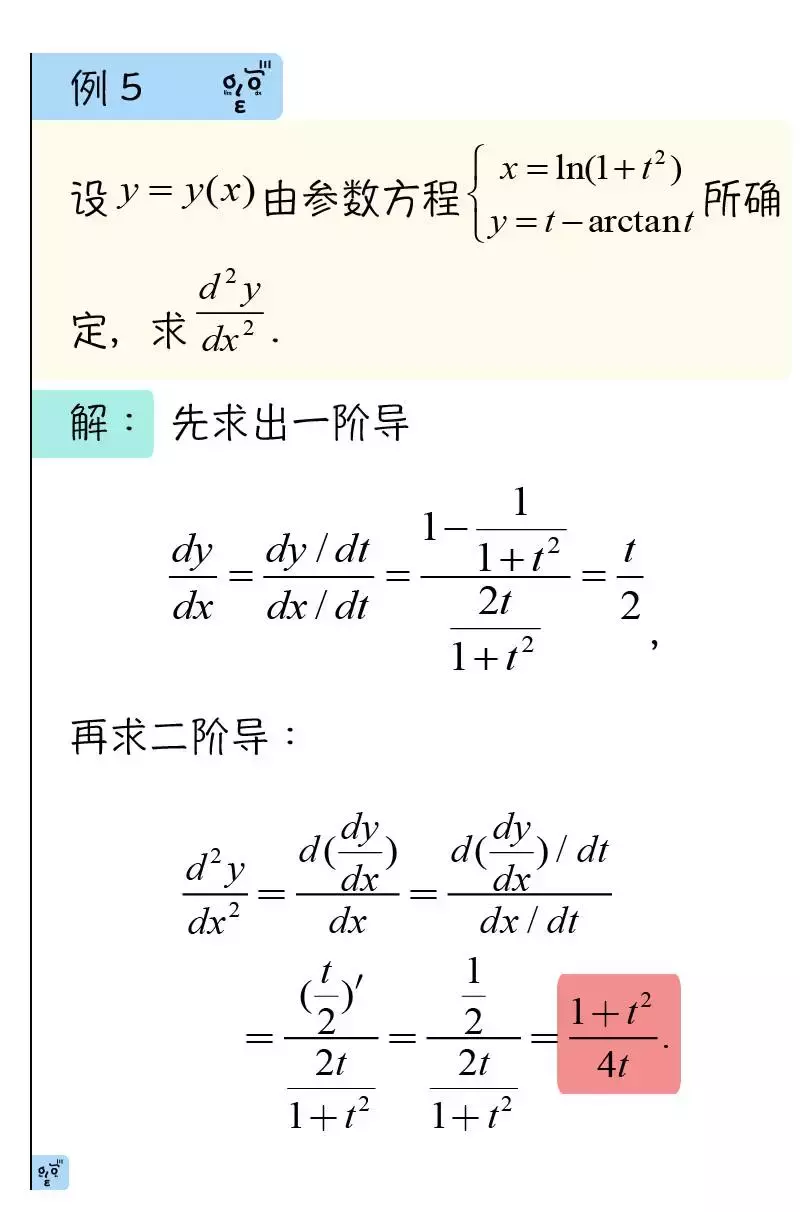本文转载自：https://www.jianshu.com/p/f3d7a8317d7b


展开全文• 不管是一元函数还是多元函数，导数、偏导数的计算都是将函数视为求导变量的一元函数求导数。微分在描述形式略有区别，但是其计算方法还是一样，只不过多元函数需要多计算几个导数而已.本文将以具体实例形式，介绍...导数与微分是微积分内容的基础，就计算来说一元函数与多元函数的导数的计算思想一致. 不管是一元函数还是多元函数，导数、偏导数的计算都是将函数视为求导变量的一元函数求导数。微分在描述形式略有区别，但是其计算方法还是一样，只不过多元函数需要多计算几个导数而已.本文将以具体实例形式，介绍线上计算具体、抽象函数的导数(偏导数)、微分与多元函数方向导数的计算方法.目录：1、一元、多元函数导数与导数值计算2、一元、多元函数高阶导数的计算3、抽象复合函数的一阶、高阶导数计算4、全微分的计算5、隐函数求一阶、二阶导数6、参数方程求一阶、二阶导数7、方向导数的计算工具：WolframAlpha计算搜索引擎位置：http://www.wolframalpha.com，打开网页直接操作，其中windows app也可以通过Windows 10应用商店下载安装！特别提示：如果使用网页版执行操作，不需要下载、安装任何软件，也不需要点任何链接，直接网页打开的那个搜索文本编辑框(如下图)输入表达式就可以了！系列推文中除特别强调外，显示的结果都能直接看到的！手机：可以直接打开网页操作，或者自行网络搜索下载安装WolframAlpha APP版本操作执行界面：网页、手机或平板等操作界面基本一致.1、一元、多元函数一阶导数与导数值的计算例1  计算以下函数的导数，并求在处的导数值：输入表达式为d/dx((x^3)cos(5x^2+e^(2x))-ln(3x^3-2x))执行后的结果如下图所示.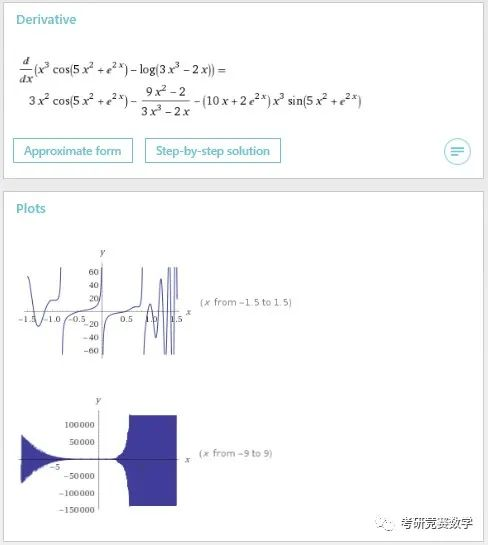结果不仅显示导数结果，也给出了函数在不同范围内的图形. 输入表达式也可以直接以更自然的语言描述形式输入，比如输入：derivative of (x^3)cos(5x^2+e^(2x))-ln(3x^3-2x)执行计算得到的结果一致.在以上两种输入的表达式后面加上where x=1，比如输入derivative of (x^3)cos(5x^2+e^(2x))-ln(3x^3-2x) where x=1执行计算后即得到导数值为例2  计算以下函数的一阶偏导数和在处的偏导数值：关于的偏导数计算输入表达式为d/dx(a sin(x^3+y^2)-(x+y^(1/2))^(1/2))执行后的结果为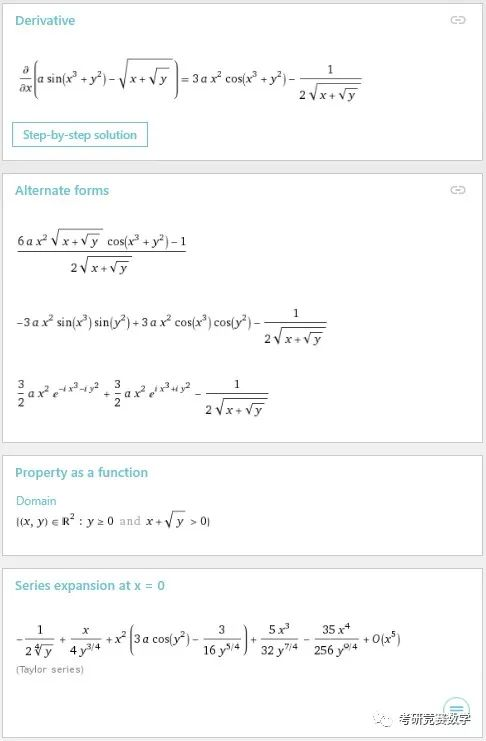结果除了最上面给出导数结果之外，在下面还以不同的形式给出了导数结果描述. 另外给出了二元函数的定义域与关于变量的带皮亚诺余项的麦克劳林公式.在以上表达式后面加上where (x,y)=(1,1)，即可得该点处的偏导数值. 即输入d/dx(a sin(x^3+y^2)-(x+y^(1/2))^(1/2)) where (x,y)=(1,1)执行计算后得到导数值为.关于的偏导数计算输入表达式只要将以上输入表达式中的求导变量改为y就可以了. 即d/dy(a sin(x^3+y^2)-(x+y^(1/2))^(1/2))执行后的结果除了导数结果不同外，其余显示内容基本一致. 其中在处的一阶导数值为.【注】  以上求导变量也可以指定为求导变量，比如输入d/da(a sin(x^3+y^2)-(x+y^(1/2))^(1/2))则计算结果为，即对变量求导，并显示导数结果图形.2、一元、多元函数高阶导数的计算例1  计算以下函数的50阶导数：输入表达式为d^50/dx^50((x^2)cosx)执行后的结果显示为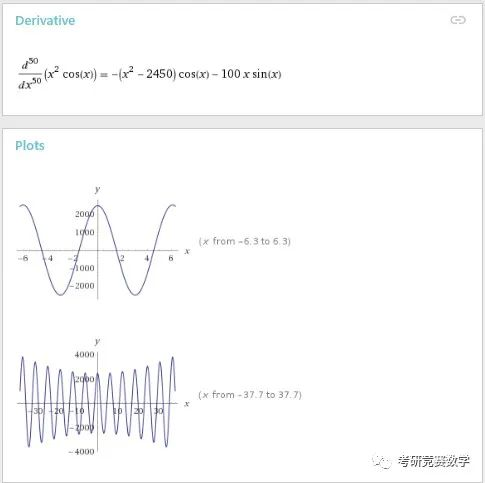例2  求以下函数关于的三阶偏导数与关于的二阶偏导数的混合高阶偏导数：输入表达式为d^3/dx^3 d^2/dy^2 ((x^2+y^2)e^(x+y))执行后显示结果.  结果除了显示偏导数外，还会显示结果曲面图、等值线图，可能的其他表达形式以及方程的根分布情况，级数展开形式，不定积分及诶过与极小值点与极小值等信息，如下图.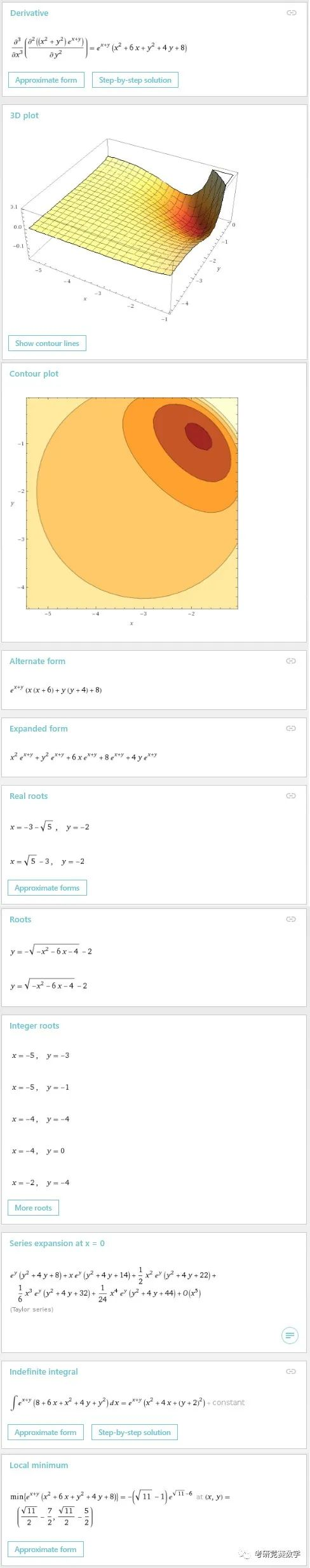3、抽象复合函数的一阶、高阶导数计算将上面具体函数求导的函数表达式换成抽象函数即可.例1  计算下列函数的一阶、二阶导数：输入表达式为d/dx (x^2)f(3x+4cosx), d^2/dx^2 (x^2)f(3x+4cosx)执行后的结果为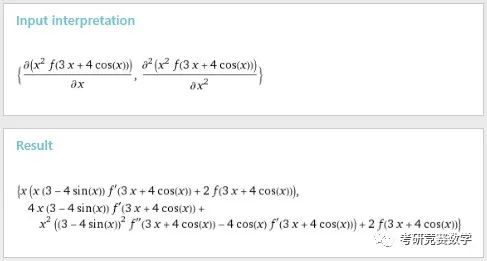由于除了外还包含其他符号，所以结果以偏导数描述形式其输入形式.例2  计算以下函数的导数：输入表达式为d^2/dx^2 f(x y, x^2-y^2), d/dx d/dy f(x y, x^2-y^2)执行后的结果为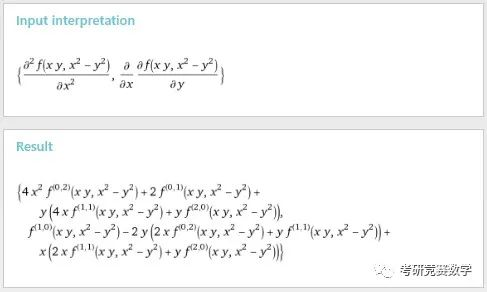4、全微分的计算由于一元函数的微分就是导数乘以自变量微分即完全可以直接归结为导数的计算，下面仅仅介绍多元函数全微分的计算方法.例  计算以下函数的全微分：直接输入表达式为derivative of a sin(x^3+y^2)-(x+y^(1/2))^(1/2)自动识别变量为, ，执行计算后的结果不仅会得到全微分表达式，也会单独列出两个偏导数. 显示结果如下：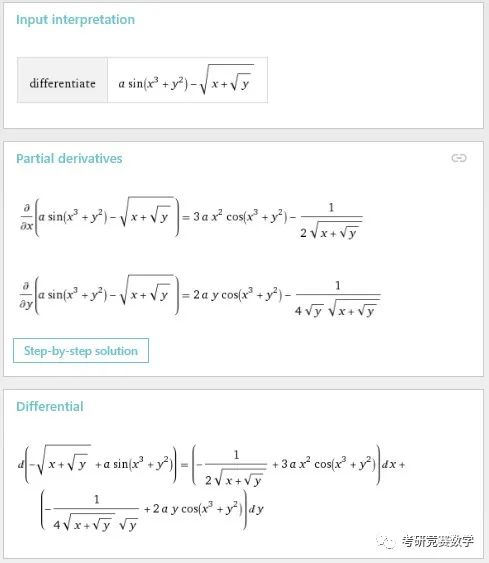其中derivative可以替换为differential. 也可以直接基于Wolfram语言，也即Mathematica中的命令来执行计算，比如输入表达式Dt(a sin(x^3+y^2)-(x+y^(1/2))^(1/2))则将表达式中的符号都识别为变量符号，执行计算得到全微分表达式. 如下图.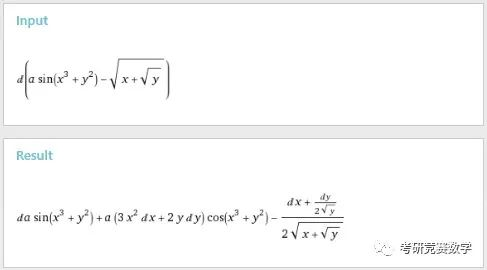只要令结果表达式中不是变量的符号，比如这里a它的微分令为0，即，得到的结果就是关于所有变量的微分表达式.5、隐函数求一阶、二阶导数例1 计算由以下方程确定的一元函数的一阶导数.参考输入表达式为derivative x^3+y^3-3a x y=0 with respect to x执行后的结果显示为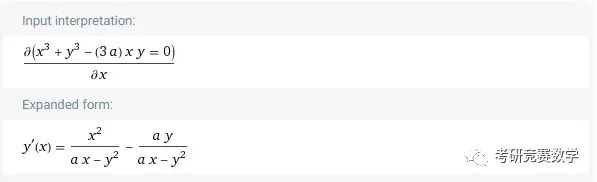例2  计算由以下方程确定的函数二阶导数：可以考虑如下参考步骤：(1) 求一阶导数derivate y(x)=tan(x+y) with respect to x执行后的结果显示为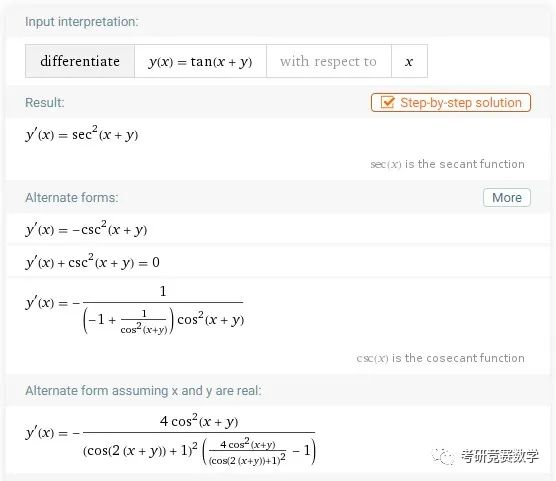(2) 对一阶导数求导数.将上面的求导等式替换为计算得到的一阶导函数，并将替换为的函数描述，如输入表达式为derivate -csc^2(x + y(x)) with respect to x将鼠标指针移动到结果显示区域，在右下角出现的选项按钮中选项中点击结果右下角的“Plain Text”，如下图.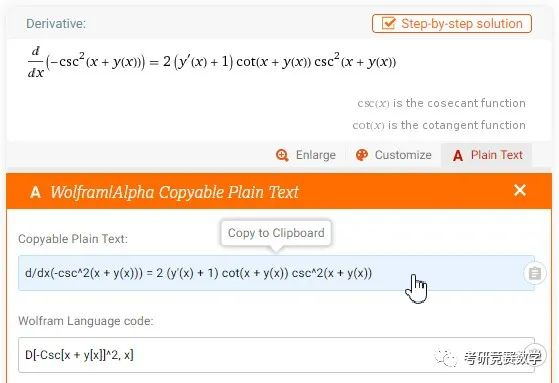在弹出结果的文本输入表达式列表中，单击第一个，则将表达式复制到剪贴板中，然后在搜索编辑框中粘贴，用等式左边的一阶导数替换右边的一阶导数，然后将等号左边的部分删除，将替换为，并在前面加上化简函数simplify，最终修改后的参考输入表达式如下simplify 2 (-csc^2(x + y) + 1) cot(x + y) csc^2(x + y)计算后得到二阶导数结果如下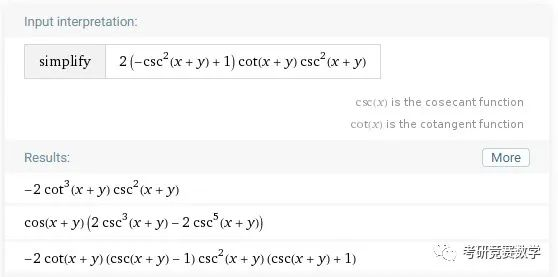6、参数方程求一阶、二阶导数参数方程求导的一阶导数直接应用公式求两个导数的比值即可，对于二阶导数则可以参照以上隐函数求二阶导数的思路，也可以直接使用求导公式计算.例  计算以下参数方程确定的函数的一阶、二阶导数：由参数方程求一阶、二阶导数公式利用公式计算一阶导数，输入表达式为(d/dt e^t sint)/(d/dt e^t cost)执行后的结果显示为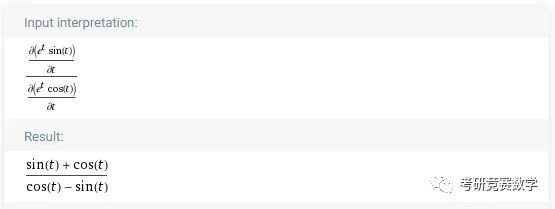除了得到一阶导数结果外，当然还会显示一阶导函数很多各种相关的描述.利用公式计算二阶导数，输入表达式为((d^2/dt^2 e^t sint)(d/dt e^t cost)-(d/dt e^t sint)(d^2/dt^2 e^t cost))/(d/dt e^t cost)^3执行后的结果显示为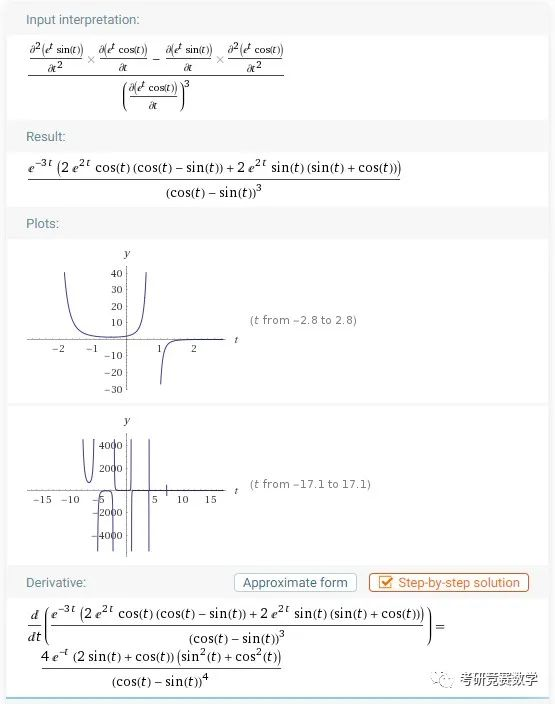7、方向导数的计算例1  计算以下函数指定方向的方向导数：输入表达式为derivative of x e^(2y)+cos(x y) in the direction (3,-4)执行后的结果显示为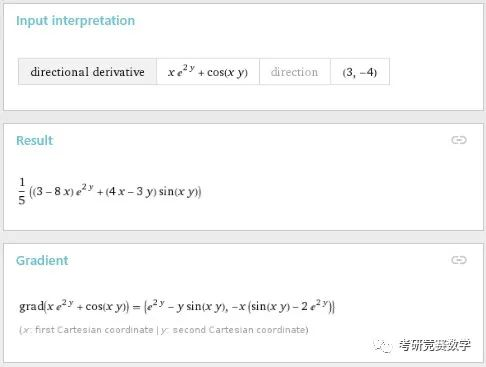不仅给出了方向导数，也给出了函数的梯度向量.例2  计算以下函数指定方向的方向导数：输入表达式为derivative of f(x,y) in the direction (a,b)执行后的结果显示为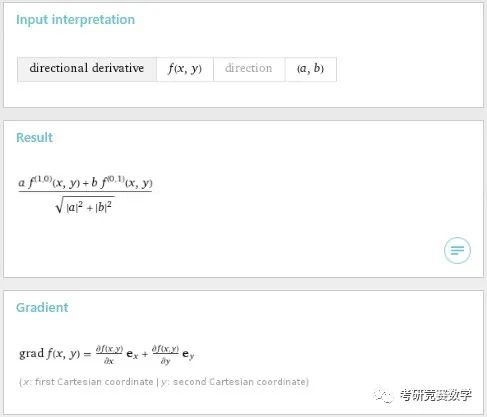例3  计算以下函数指定方向和点处的方向导数：输入表达式为derivative 3x^2+2y^2+z^2 in direction (-2,-2,1) at point (1,2,3)执行后的结果显示为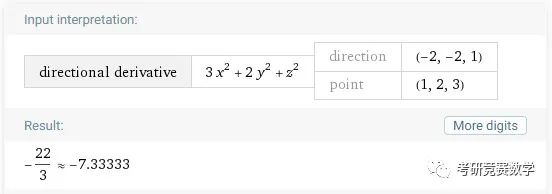当然以上计算也可以直接依据求偏导数与方向导数计算公式，逐步计算代入得到结果.微信公众号：考研竞赛数学(ID: xwmath)大学数学公共基础课程分享交流平台！支持咱号请点赞分享！↓↓↓点查看更多相关内容

展开全文• 不管是一元函数还是多元函数，导数、偏导数的计算都是将函数视为求导变量的一元函数求导数。微分在描述形式略有区别，但是其计算方法还是一样，只不过多元函数需要多计算几个导数而已.本文将以具体实例形式，介绍...
• 隐函数求导 参数方程求导 隐函数 顾名思义，隐函数可以理解为隐藏的函数。自打我们学习函数以来，大部分的函数都是这样子 自变量和因变量的值都是分散在=号两侧，楚河汉界，泾渭分明。也就是说y和x的位置永远是相异...
• 本实验涉及微积分和微分方程。通过实验复习曲线的参数方程及其求导、复合函数微商法、微分方程的建立及求解和某些二次曲面等知识；另外通过实际问题介绍平面单参数曲线族包络线的概念及其应用。
• 另一方面，阿基米德螺旋线参数方程求导： 所以，可以看到，换算至笛卡尔坐标导数不是常数，而和角度有关系，这个很好理解， 切线斜率一直在变化。那么，我们看看一般意义下的推导，极坐标所有曲线一定...
• 对于参数方程求导，有些同学有疑问，而且按照自己的思路越想越想不通，然后开始怀疑高数，进而怀疑智商，最后开始怀疑人生，内心的默默念着我。。。太。。。难。。。了！！！其实很简单，把思路捋顺就行了。 首先...
• 比如y-x+1=0这种可以简单的进行显示化的函数，可以变化成y=x-1 2… 当函数显示化比较困难时，可以等式两边对x求导，将y看做是关于x的函数 例题 例如ex+y=x2+y+1 例2 例3 例4 例5 由参数方程确定的函数求导 ...
• 095: 复习习题 求导题型 Case1：显函数求导；Case2：隐函数求导；Case3：参数方程确定的函数函数 高等数学
• 参数函数求导 1. 利用莱布尼茨定理求高阶导 只看两点： 1、常用导数的高阶公式 2、例题 例题： 2.隐函数求导 这种方程里面y是x的函数，但是不显性。 例题1 设 y=y(x),y2−2xy+9=0y = y(x), y^2 - 2xy +9 = 0y=y(x)...
• 关键词 本文论述复合函数求导法则证明的 另 一种方法 , 并用 此 方 法论证参数方程求导 复合函数 求导法则 证明 众所周知 , 复合函数求导法则的经典证明方法和参数方程求导法则 的经典证明一直延用至今 。...
• ∴ u = g(x) 在 x 处连续，lim Δx→0 Δu = 0 联合 ② 式可知 lim Δx→0 α = 0 ∴ ④ = f'(u) * g'(x) = f'(g(x)) * g'(x) ch5-8 微分及其运算、隐函数与参数方程函数求导 概念与定义 联立符号 - 本笔记中联立...可微偏导数一定存在
• 比如，反函数求导，隐函数求导，参数方程求导的方法。再简单讨论一下高阶导数的概念。 先看看考纲对这一块的要求： 3. 了解高阶导数的概念，会求简单函数的高阶导数． 4. 会求分段函数的导数，会求隐函数和由参数...高等数学
• 2.参数方程求导 syms t; f1=t^4; f2=4*t; diff(f2)/diff(f1) 3.隐函数求导（等式两边放一起即可转变为隐函数） syms x y; f=atan(y/x)-log(sqrt(x^2+y^2));%隐函数求导 xy=-diff(f,x)/diff(...
• 关于一元函数微分学，专升本数学考试要求包括：(一)导数与微分1．理解导数的概念及其几何意义，了解左导数与右导数的定义，理解函数的可导性与连续性的关系，会用定义求函数在...掌握对数求导法与参数方程求导法。...
• 关于一元函数微分学，专升本数学考试要求包括：(一)导数与微分1．理解导数的概念及其几何意义，了解左导数与右导数的定义，理解函数的可导性与连续性的关系，会用定义求函数在...掌握对数求导法与参数方程求导法。...
• 关于一元函数微分学，专升本数学考试要求包括：(一)导数与微分1．理解导数的概念及其几何意义，了解左导数与右导数的定义，理解函数的可导性与连续性的关系，会用定义求函数在...掌握对数求导法与参数方程求导法。...
• 01 求高阶导数的方法+视频课程上课手稿02 求反函数二阶导的方法+视频课程上课手稿03求参数方程二阶导数的方法+视频课程上课手稿04 求分段函数导数的方法+视频课程上课手稿05求变限积分函数导数的方法+视频课程上课...
• 一、隐函数的导数 1.1、隐函数与显函数 1.2、方程确定函数 1.3、隐函数的显化，隐函数的导数 1.4、例题 1.4.1、例1 ...二、由参数方程所确定的函数的导数 2.1、由抛物运动引出 （4-2）将t消去有： 2...
• 一、常见函数的高阶导数 7是莱布尼茨公式 二、隐函数求导 注意对数求导法 隐函数的高阶导数就是对方程两边多求几次导，然后把低价导数代入等式。 三、参数方程求导 ...
• 关于高等数学参数方程二阶可导的公式推导 理解为在一阶导数的基础上，对x再次求导。 个人理解：d(dy/dx)/dx 整体可以看作先对t求导，再令t对x求导；而括号中的dy/dx则是g’(t)/f’(t)；得出第一个等号后的式子。 ...
• (2)参数方程求导 题型框架 题型1：考定义 可导必连续 可导有左导=右导 深刻理解limlimlim下的那个趋于0的变量 讨论是否可导就是讨论某个极限是否存在 题型2：各种求导 隐函数求导 参数方程求导 分段函数求导 ...
• 在高中物理曲线运动和后面万有引力两章中都涉及到匀速圆周运动。...以下是比较正经的，按照高中数学范围的，列参数方程，二次求导。如同这样 以x轴正半轴为时间0点，逆时针为正反向。 直角坐标方程： 极坐标形式： ...
• matlab实现参数方程求导 具体描述见下面网址： https://blog.csdn.net/weixin_43964993/article/details/107863569matlab 微积分...